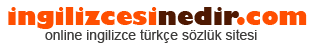ingilizce türkçe sözlük sitesine hoşgeldiniz.| Ana Sayfaİngilizce Türkçe Sözlükte Ara: Türkçe->İngilizce İngilizce->Türkçe * Aramak istediğiniz kelimeyi yazıp dil seçimini seçtikten sonra kelimenin anlamını öğrenebilirsiniz.

Kelime Anlamı

## Türkçeden İngilizceye Çeviri Sonucu

### momentum Anlamı

• The flow of activities and the pace of teaching and learning maintained in a classroom. 'the effect of an impelling force, suddenly and momentarily communicated'. an impelling force or strength; 'the car's momentum carried it off the road'. the product of a body's mass and its velocity; 'the momentum of the particles was deduced from meteoritic velocities'.
• Momentum is the most basic concept in oscillator analysis Momentum is the rate of change at which the market is rising or falling.
• Like in physics, a market in motion tends to stay in motion unless it goes too far too fast Momentum indicators signal overbought or oversold when they move to extreme levels.
• Mass of body multiplied by its velocity.
• Linear momentum, mv.
• We will know that we have 'momentum' when many of the significant problems and issues that the church faces initially have been dealt with and precedents set so that the forward energy of the church is unleashed and focussed on expansion.
• Designed to measure the rate of price change, not the actual price level Consists of the net difference between the current closing price and the oldest closing price from a predetermined period The Momentum indicator can be used as either a trend-following oscillator similar to the MACD or as a leading indicator.
• The rate of acceleration of an economic, price or volume movement An economy with strong growth that is likely to continue is said to have momentum.
• Quantity of motion Linear momentum is the quantity obtained by multiplying the mass of a body by its linear speed Angular momentum is the quantity obtained by multiplying the moment of inertia of a body by its angular speed The momentum of a system of particles is given by the sum of the momentums of the individual particles which make up the system or by the product of the total mass of the system and the velocity of the center of gravity of the system The momentum of a continuous medium is given by the integral of the velocity over the mass of the medium or by the product of the total mass of the medium and the velocity of the center of gravity of the medium.
• Having the leverage necessary to control an opponent's moves through the use of threats Creating momentum is the most widely-used, and most effective, strategy for the game This situation is also called initiative and tempo.
• The rate of change of a share price Hence momentum traders follow rapidly moving markets.
• Mass times velocity; a quantity that determines the potential force that an object can impart to another object by collision.
• Inertia where the object is in motion If the object's speed is much lower than the speed of light, momentum is mass times velocity.
• The combination of an object's mass and its velocity A massive object going at a high velocity has a large momentum.
• The property of a moving object equal to its mass times its velocity. the product of the mass and velocity of a body-a vector quantity.
• The product of the mass and velocity of a moving body.
• The product of an object's mass and its velocity.
• In technical analysis, the relative change in price over a specific time interval Often equated with speed or velocity and considered in terms of relative strength.
• Momentum is a property of any moving object For a slow moving object it is given by the mass times the velocity of the object For an object moving at close to the speed of light this definition gets modified The total momentum is a conserved quantity in any process Physicists use the letter p to represent momentum, presumably because m was already used for mass, n for number, and o is too much like zero.
• Essential element, or constituent element. the product of a body's mass and its velocity; 'the momentum of the particles was deduced from meteoritic velocities' an impelling force or strength; 'the car's momentum carried it off the road'.
• The quantity of motion in a moving body, being always proportioned to the quantity of matter multiplied into the velocity; impetus.
Rastgele Türkçe Kelimeler